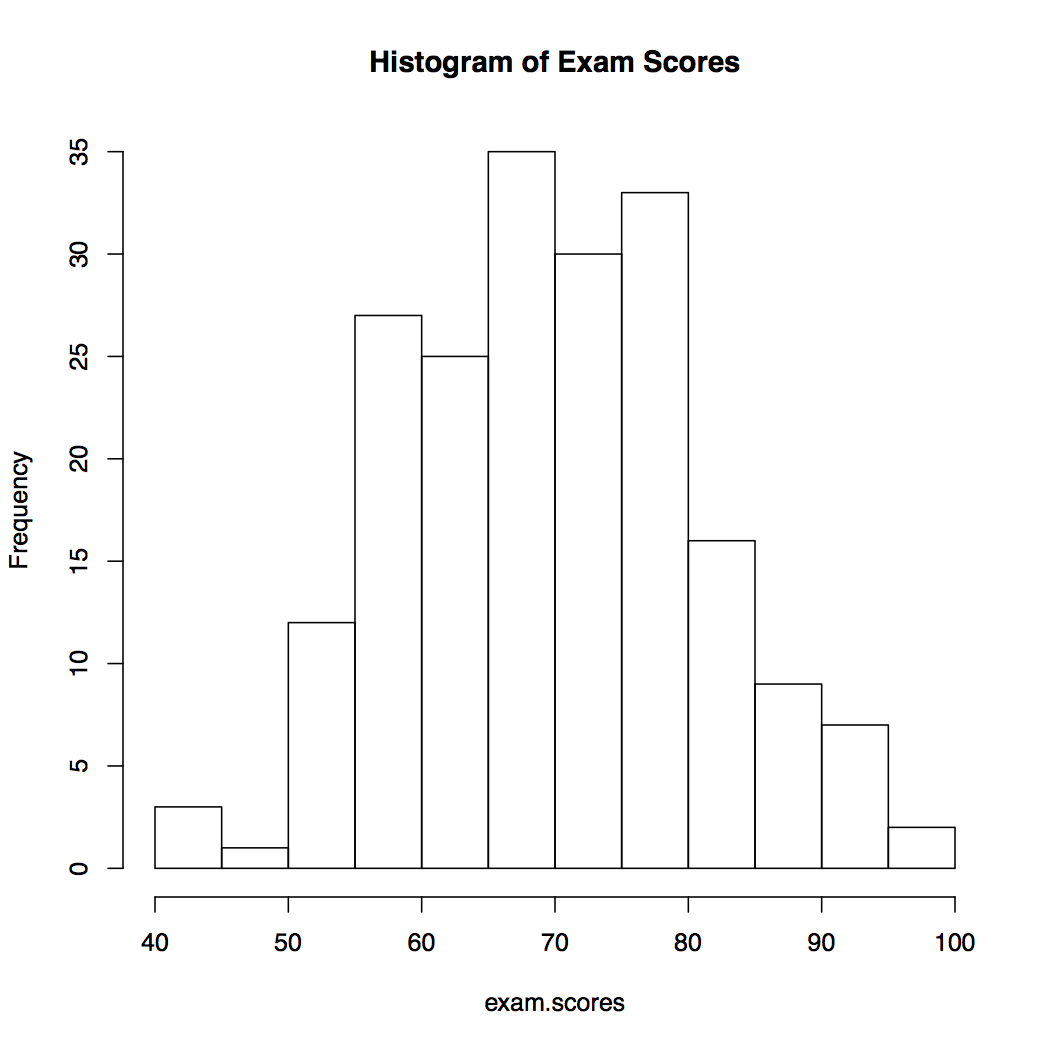# 4.1 - Sampling Distribution of the Sample Mean

Let’s put some numbers into Ellie’s example.

Note! The sampling method is done without replacement.

## Sample Means with a Small Population: Runner’s MIleage Section

In this example, the population is the mileage of six runners. Ellie is going to try to guess the true average mileage of the six runners by taking a random sample without replacement from the population.

Mileage A B C D E F
19 14 15 9 10 17

Since we know the miles from the population, we can find the population mean.

$$\mu=\dfrac{19+14+15+9+10+17}{6}=14$$ miles

To demonstrate the sampling distribution, let’s start with obtaining all of the possible samples of size $$n=2$$ from the populations, sampling without replacement. The table below show all the possible samples, the weights for the chosen runners the sample mean and the probability of obtaining each sample. Since we are drawing at random, each sample will have the same probability of being chosen.

View Full Table

 Sample Mileage $$\boldsymbol{\bar{x}}$$ Probability A, B 19, 14 16.5 $$\frac{1}{15}$$ A, C 19, 15 17.0 $$\frac{1}{15}$$ A, D 19, 9 14.0 $$\frac{1}{15}$$ A, E 19, 10 14.5 $$\frac{1}{15}$$ A, F 19, 17 18.0 $$\frac{1}{15}$$ B, C 14, 15 14.5 $$\frac{1}{15}$$ B, D 14, 9 11.5 $$\frac{1}{15}$$ B, E 14, 10 12.0 $$\frac{1}{15}$$ B, F 14, 17 15.5 $$\frac{1}{15}$$ C, D 15, 9 12.0 $$\frac{1}{15}$$ C, E 15, 10 12.5 $$\frac{1}{15}$$ C, F 15, 17 16.0 $$\frac{1}{15}$$ D, E 9, 10 9.5 $$\frac{1}{15}$$ D, F 9, 17 13.0 $$\frac{1}{15}$$ E, F 10, 17 13.5 $$\frac{1}{15}$$

We can combine all of the values and create a table of the possible values and their respective probabilities.

 $$\boldsymbol{\bar{x}}$$ 9.5 11.5 12.0 12.5 13.0 13.5 14.0 14.5 15.5 16.0 16.5 17.0 18.0 Probability $$\frac{1}{15}$$ $$\frac{1}{15}$$ $$\frac{2}{15}$$ $$\frac{1}{15}$$ $$\frac{1}{15}$$ $$\frac{1}{15}$$ $$\frac{1}{15}$$ $$\frac{2}{15}$$ $$\frac{1}{15}$$ $$\frac{1}{15}$$ $$\frac{1}{15}$$ $$\frac{1}{15}$$ $$\frac{1}{15}$$

The table is the probability table for the sample mean and it is the sampling distribution of the sample mean mileage of the runners when the sample size is 2. It is also worth noting that the sum of all the probabilities equals 1. It might be helpful to graph these values.

One can see that the chance that the sample mean is exactly the population mean is only 1 in 15, very small. (In some other examples, it may happen that the sample mean can never be the same value as the population mean.) When using the sample mean to estimate the population mean, some possible error will be involved since the sample mean is random.

Now that we have the sampling distribution of the sample mean, we can calculate the mean of all the sample means. In other words, we can find the mean (or expected value) of all the possible $$\bar{x}$$’s.

The mean of the sample means is

$$\mu_\bar{x}=\sum \bar{x}_{i}f(\bar{x}_i)=9.5\left(\frac{1}{15}\right)+11.5\left(\frac{1}{15}\right)+12\left(\frac{2}{15}\right)\\+12.5\left(\frac{1}{15}\right)+13\left(\frac{1}{15}\right)+13.5\left(\frac{1}{15}\right)+14\left(\frac{1}{15}\right)\\+14.5\left(\frac{2}{15}\right)+15.5\left(\frac{1}{15}\right)+16\left(\frac{1}{15}\right)+16.5\left(\frac{1}{15}\right)\\+17\left(\frac{1}{15}\right)+18\left(\frac{1}{15}\right)=14$$

Even though each sample may give you an answer involving some error, the expected value is right at the target: exactly the population mean. In other words, if one does the experiment over and over again, the overall average of the sample mean is exactly the population mean.

Now, let's do the same thing as above but with sample size $$n=5$$

 Sample Mileage $$\boldsymbol{\bar{x}}$$ Probability A, B, C, D, E 19, 14, 15, 9, 10 13.4 1/6 A, B, C, D, F 19, 14, 15, 9, 17 14.8 1/6 A, B, C, E, F 19, 14, 15, 10, 17 15.0 1/6 A, B, D, E, F 19, 14, 9, 10, 17 13.8 1/6 A, C, D, E, F 19, 15, 9, 10, 17 14.0 1/6 B, C, D, E, F 14, 15, 9, 10, 17 13.0 1/6

The sampling distribution is:

 $$\boldsymbol{\bar{x}}$$ 13.0 13.4 13.8 14.0 14.8 15.0 Probability 1/6 1/6 1/6 1/6 1/6 1/6

The mean of the sample means is...

$$\mu=(\dfrac{1}{6})(13+13.4+13.8+14.0+14.8+15.0)=14$$ miles

The following dot plots show the distribution of the sample means corresponding to sample sizes of $$n=2$$ and of $$n=5$$.

Again, we see that using the sample mean to estimate population mean involves sampling error. However, the error with a sample of size $$n=5$$ is on the average smaller than with a sample of size$$n= 2$$.

## Sampling Error and Size Section

Sampling Error
The error resulting from using a sample characteristic to estimate a population characteristic.

Sample size and sampling error: As the dot plots above show, the possible sample means cluster more closely around the population mean as the sample size increases. Thus, the possible sampling error decreases as sample size increases.

What happens when the population is not small?

## Sample Means with Large Samples Section

An instructor of an introduction to statistics course has 200 students. The scores out of 100 points are shown in the histogram.The population mean is $$\mu=69.77$$ and the population standard deviation is $$\sigma=10.9$$.

Let's demonstrate the samping distribution of the sample means using the StatKey website. The first video will demonstrate the sampling distribution of the sample mean when n = 10 for the exam scores data. The second video will show the same data but with samples of n = 30.

You should start to see some patterns. The mean of the sampling distribution is very close to the population mean. The standard deviation of the sampling distribution is smaller than the standard deviation of the population.

In the examples so far, we were given the population and sampled from that population.

What happens when we do not have the population to sample from? What happens when all that we are given is the sample? Fortunately, we can use some theory to help us. The mathematical details of the theory are beyond the scope of this course but the results are presented in this lesson.

In the next two sections, we will discuss the sampling distribution of the sample mean when the population is Normally distributed and when it is not.